# Allele

In genetics, an allele (pronounced al-eel or al-e-ul) is any one of a number of viable DNA (deoxyribonucleic acid) codings occupying a given locus (position) on a chromosome. Usually alleles are DNA sequences that code for a gene, but sometimes the term is used to refer to a non-gene sequence.

An individual's genotype for that gene is the set of alleles it happens to possess. In a diploid organism, one that has two copies of each chromosome, two alleles make up the individual's genotype.

An example is the gene for blossom color in many species of flower—a single gene controls the color of the petals, but there may be several different versions (or alleles) of the gene. One version might result in red petals, while another might result in white petals. The resulting color of an individual flower will depend on which two alleles it possesses for the gene and how the two interact.

## Contents

The study of genetics and the identification of alleles has been of tremendous importance for medicine and understanding living organisms and holds great promise for improved clinical practice.

## Introduction

Diploid organisms, such as humans, have paired, homologous chromosomes in their somatic cells (body cells, not germ cells), and these contain two copies of each gene. An organism in which the two copies of the gene are identical—that is, have the same allele—is called homozygous for that gene. An organism which has two different alleles of the gene is called heterozygous.

Phenotypes (the expressed characteristics) associated with a certain allele can sometimes be dominant or recessive, but often they are neither. A dominant phenotype will be expressed when at least one allele of its associated type is present, whereas a recessive phenotype will only be expressed when both alleles are of its associated type.

However, there are exceptions to the way heterozygotes express themselves in the phenotype. One exception is incomplete dominance (sometimes called blending inheritance) when alleles blend their traits in the phenotype. An example of this would be seen if, when crossing Antirrhinums—flowers with incompletely dominant "red" and "white" alleles for petal color—the resulting offspring had pink petals.

Another exception is co-dominance, where both alleles are active and both traits are expressed at the same time; for example, both red and white petals in the same bloom, or red and white flowers on the same plant. Codominance is also apparent in human blood types. A person with one "A" blood type allele and one "B" blood type allele would have a blood type of "AB."

Sickle-cell disease is an example of a single-allele disorder that operates in a co-dominant system (Towle 1989). Dominant allele A produces normal hemoglobin and co-dominant allele A' codes for abnormal hemoglobin (resulting in sickle-shaped erythrocytes). AA individuals have normal hemoglobin and erythrocytes, AA' individuals have both normal and abnormal hemoglobin and intermediate shaped cells, and A'A' individuals have sickle cells, which clump together, clog the capillaries, cause pain, and impair oxygen flow (Towle 1989).

A wild type allele is an allele that is considered to be "normal" for the organism in question, as opposed to a mutant allele, which is usually a relatively new modification.

Note that with the advent of neutral genetic markers, the term 'allele' is now often used to refer to DNA sequence variants in non-functional, or junk DNA. For example, allele frequency tables are often presented for genetic markers, such as the DYS markers.

## Equations

There are two equations for the frequency of two alleles of a given gene (see Hardy-Weinberg principle).

Equation 1: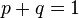$p+q=1$,

Equation 2: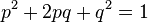$p^2+2pq+q^2=1$

where$p$ is the frequency of one allele and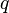$q$ is the frequency of the other allele. Under appropriate conditions, subject to numerous limitations regarding the applicability of the Hardy-Weinberg principle,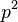$p^2$ is the population fraction that is homozygous for the$p$ allele,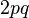$2pq$ is the frequency of heterozygotes, and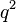$q^2$ is the population fraction that is homozygous for the$q$ allele.

Natural selection is considered to be a factor that can act on$p$ and$q$ in Equation 1, and obviously affect the frequency of alleles seen in Equation 2.

Equation 2 is a consequence of Equation 1, obtained by squaring both sides and applying the binomial theorem to the left-hand side. Conversely,$p^2+2pq+q^2=1$ implies$p+q=1$ since$p$ and$q$ are positive numbers.

The following equation (commonly termed the Lee equation) can be used to calculate the number of possible genotypes in a diploid organism for a specific gene with a given number of alleles.

G = 0.5a^2 + 0.5a

where 'a' is the number of different alleles for the gene being dealt with and 'G' is the number of possible genotypes.

For example, the human ABO blood group gene has three alleles; A (for blood group A), B (for blood group B), and i (for blood group O). As such, using the equation, the number of possible genotypes a human may have with respect to the ABO gene are 6 (AA, Ai, AB, BB, Bi, ii). Note that the equation does not in any way calculate the number of possible phenotypes. Such an equation would be quite impossible, as the number of possible phenotypes varies among different genes and their alleles. For example, in a diploid heterozygote some genotypes may show complete dominance, incomplete dominance, and so forth, depending of the gene involved.